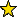# SymbolicPowers -- symbolic powers of ideals

## Description

This package gives the ability to compute symbolic powers, and related invariants, of ideals in a polynomial ring or a quotient of a polynomial ring. For example, in the context of the default behavior, symbolicPower assumes the following definition of the symbolic power of an ideal $I$, $$I^{(n)} = \cap_{p \in Ass(R/I)}(I^nR_p \cap R ),$$ as defined by M. Hochster and C. Huneke.

Alternatively, as defined in Villarreal, symbolicPower has the option to restrict to minimal primes versus use all associated primes with UseMinimalPrimes. In particular, the symbolic power of an ideal $I$ is defined as $$I^{(n)} = \cap_{p \in Min(R/I)}(I^nR_p \cap R ),$$ where $Min(R/I)$ is the set of minimal primes in $I$,

• M. Hochster and C. Huneke. Comparison of symbolic and ordinary powers of ideals. Invent. Math. 147 (2002), no. 2, 349–369.
• R. Villarreal. Monomial algebras. Second edition. Monographs and Research Notes in Mathematics. CRC Press, Boca Raton, FL, 2015. xviii+686 pp. ISBN: 978-1-4822-3469-5.
• Hailong Dao, Alessandro De Stefani, Eloísa Grifo, Craig Huneke, and Luis Núñez-Betancourt. Symbolic powers of ideals.in Singularities and foliations. Geometry, topology and applications, pp. 387-432, Springer Proc. Math. Stat., 222, Springer, Cham, 2018. See https://arxiv.org/abs/1708.03010.

## Contributors

The following people have generously contributed code or worked on our code at various Macaulay2 workshops.
• Ben Drabkin
• Andrew Conner
• Alexandra Seceleanu
• Branden Stone
• Xuehua (Diana) Zhong

## CertificationVersion 2.0 of this package was accepted for publication in volume 9 of The Journal of Software for Algebra and Geometry on 20 May 2019, in the article Calculations involving symbolic powers. That version can be obtained from the journal or from the Macaulay2 source code repository.

## Version

This documentation describes version 2.0 of SymbolicPowers.

## Source code

The source code from which this documentation is derived is in the file SymbolicPowers.m2.

## Exports

• Functions and commands
• Methods
• "assPrimesHeight(Ideal)" -- see assPrimesHeight -- The heights of all associated primes
• "asymptoticRegularity(Ideal)" -- see asymptoticRegularity -- approximates the asymptotic regularity
• "bigHeight(Ideal)" -- see bigHeight -- computes the big height of an ideal
• "containmentProblem(Ideal,ZZ)" -- see containmentProblem -- computes the smallest symbolic power contained in a power of an ideal.
• "isKonig(Ideal)" -- see isKonig -- determines if a given square-free ideal is Konig.
• "isPacked(Ideal)" -- see isPacked -- determines if a given square-free ideal is packed.
• "isSymbolicEqualOrdinary(Ideal,ZZ)" -- see isSymbolicEqualOrdinary -- tests if symbolic power is equal to ordinary power
• "isSymbPowerContainedinPower(Ideal,ZZ,ZZ)" -- see isSymbPowerContainedinPower -- tests if the m-th symbolic power an ideal is contained the n-th power
• "joinIdeals(Ideal,Ideal)" -- see joinIdeals -- Computes the join of the given ideals
• "lowerBoundResurgence(Ideal)" -- see lowerBoundResurgence -- computes a lower bound for the resurgence of a given ideal.
• "minDegreeSymbPower(Ideal,ZZ)" -- see minDegreeSymbPower -- returns the minimal degree of a given symbolic power of an ideal.
• "minimalPart(Ideal)" -- see minimalPart -- intersection of the minimal components
• "noPackedAllSubs(Ideal)" -- see noPackedAllSubs -- finds all substitutions of variables by 1 and/or 0 for which ideal is not Konig.
• "noPackedSub(Ideal)" -- see noPackedSub -- finds a substitution of variables by 1 and/or 0 for which an ideal is not Konig.
• "squarefreeGens(Ideal)" -- see squarefreeGens -- returns all square-free monomials in a minimal generating set of the given ideal.
• "squarefreeInCodim(Ideal)" -- see squarefreeInCodim -- finds square-fee monomials in ideal raised to the power of the codimension.
• "symbolicDefect(Ideal,ZZ)" -- see symbolicDefect -- computes the symbolic defect of an ideal
• "symbolicPolyhedron(Ideal)" -- see symbolicPolyhedron -- computes the symbolic polyhedron for a monomial ideal.
• "symbolicPolyhedron(MonomialIdeal)" -- see symbolicPolyhedron -- computes the symbolic polyhedron for a monomial ideal.
• "symbolicPower(Ideal,ZZ)" -- see symbolicPower -- computes the symbolic power of an ideal.
• "symbolicPowerJoin(Ideal,ZZ)" -- see symbolicPowerJoin -- computes the symbolic power of the prime ideal using join of ideals.
• "symbPowerPrimePosChar(Ideal,ZZ)" -- see symbPowerPrimePosChar
• "waldschmidt(Ideal)" -- see waldschmidt -- computes the Waldschmidt constant for a homogeneous ideal.
• "waldschmidt(MonomialIdeal)" -- see waldschmidt -- computes the Waldschmidt constant for a homogeneous ideal.
• Symbols
• CIPrimes -- compute the symbolic power by taking the intersection of the powers of the primary components
• InSymbolic -- an optional parameter used in containmentProblem.
• SampleSize -- optional parameter used for approximating asymptotic invariants that are defined as limits.
• UseMinimalPrimes -- an option to only use minimal primes to calculate symbolic powers
• UseWaldschmidt -- optional input for computing a lower bound for the resurgence of a given ideal.

## For the programmer

The object SymbolicPowers is .